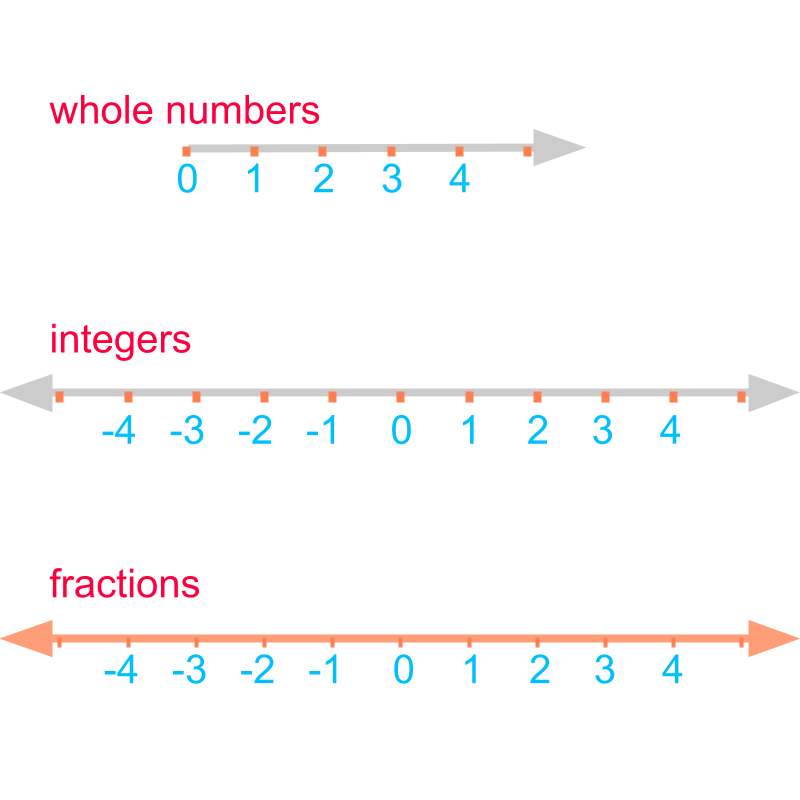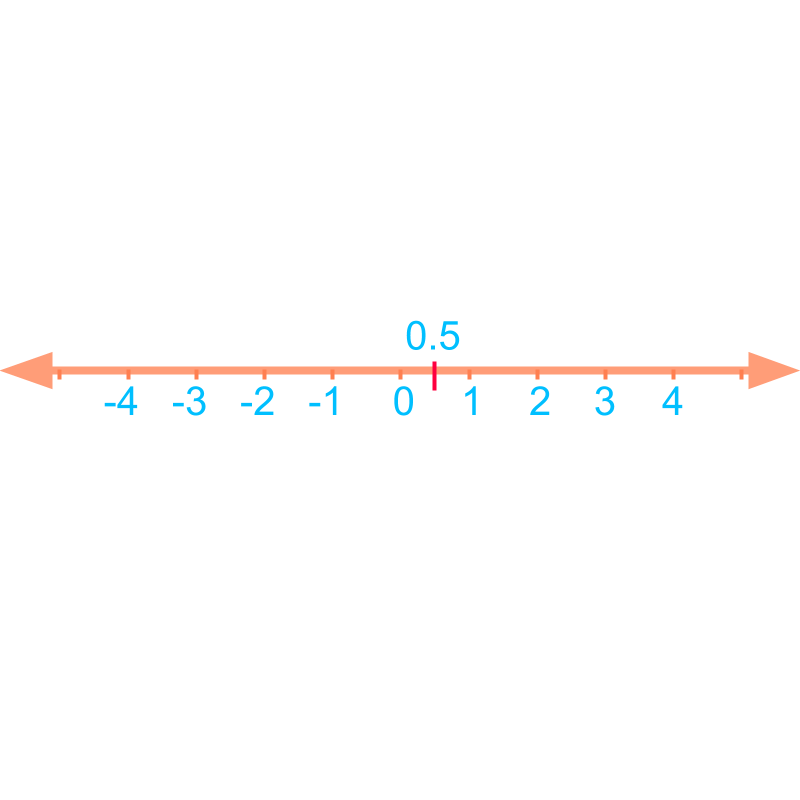maths > decimals

Decimals : Expanded form and number-line

what you'll learn...

overview

This page covers expanded form of decimals and simple overview of placing decimals in number-line.

Expanded form provides the place value of each digits of the number.
Eg: $12.34=10+2+3/10+4/100$$12.34 = 10 + 2 + 3 / 10 + 4 / 100$

Decimals are placed with the integers and maintains the ordinal property. Thus the decimals are placed in the number-line.

warm up to learn

We studied expanded forms in whole numbers. The number $734$$734$ in expanded form is "$700$$700$$+30+4$$+ 30 + 4$".

The word 'expand' means: to make something large by giving more details.

expanded form of decimal

The expanded form of $32.625$$32.625$is "$30+2+6/10+2/100+5/1000$$30 + 2 + 6 / 10 + 2 / 100 + 5 / 1000$".

Expanded form : provides the place value of each digits of the number.
Eg: $12.34=10+2+3/10+4/100$$12.34 = 10 + 2 + 3 / 10 + 4 / 100$

What is the expanded form of $0.0023$$0.0023$?
The answer is "$2/1000+3/10000$$2 / 1000 + 3 / 10000$".

ordered sequence

In whole numbers, we learned that the ordinal-property of the numbers is defined by $0<1<2<3<\cdots$$0 < 1 < 2 < 3 < \cdots$.

In integers, we learned that the ordinal-property of the numbers is extended to $\cdots <-3<-2<-1<$$\cdots < - 3 < - 2 < - 1 <$$0<1<2<3<\cdots$$0 < 1 < 2 < 3 < \cdots$.

In fractions, we learned that the fractions are placed within the integer numbers and maintains the ordinal property.

Decimals do have ordinal property -- ordinal property for decimals is same as that of fractions.The ordinal property is best captured by the number-line.

•  Number-line of whole numbers starts from $0$$0$ and extends in one direction. It consists of points at positions $0,1,2,\cdots$$0 , 1 , 2 , \cdots$.

•  Number-line of integers extends in both the directions. It consists of points at positions $\cdots ,-2,-1,0,1,2,\cdots$$\cdots , - 2 , - 1 , 0 , 1 , 2 , \cdots$

•  Number-line of fractions is same as that of integers, and fractions are placed on points on the line segments between the integers. "the number-line of decimals is same as that of fractions as decimals are an alternate representation of fractions"Consider the number line of decimals. Where is the decimal $0.5$$0.5$ located in the number-line?

"$0.5$$0.5$ is between integers $0$$0$ and $1$$1$". The line-segment between $0$$0$ and $1$$1$ is split into $10$$10$ equal pieces. $0.5$$0.5$ is at the position of $5$$5$th piece as shown in the figure.

Consider the number line of decimals.

The decimal $2.34$$2.34$ located in the number-line is located between integers $2$$2$ and $3$$3$ at $34$$34$th position of $100$$100$ pieces". The line-segment between $2$$2$ and $3$$3$ is split into $100$$100$ equal pieces. $2.34$$2.34$ is at the position of $34$$34$th piece.

summary

Expanded form : provides the place value of each digits of the number.
Eg: $12.34=10+2+3/10+4/100$$12.34 = 10 + 2 + 3 / 10 + 4 / 100$

Decimals do have ordinal property -- ordinal property for decimals is same as that of fractions.

Oridnal property of integers is given by $\cdots <-3<-2<-1<$$\cdots < - 3 < - 2 < - 1 <$$0<1<2<3<\cdots$$0 < 1 < 2 < 3 < \cdots$

The fractions are placed within the integers and maintains the ordinal property.

Similarly, decimals are placed with the integers and maintains the ordinal property.

Outline